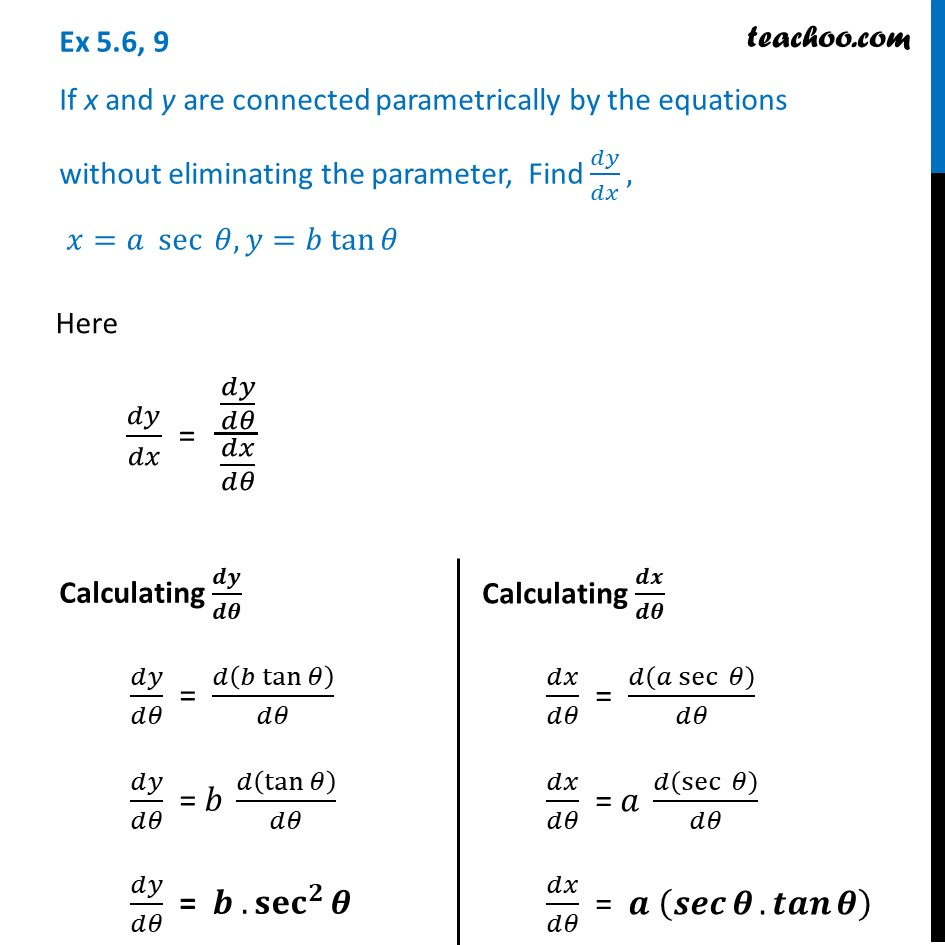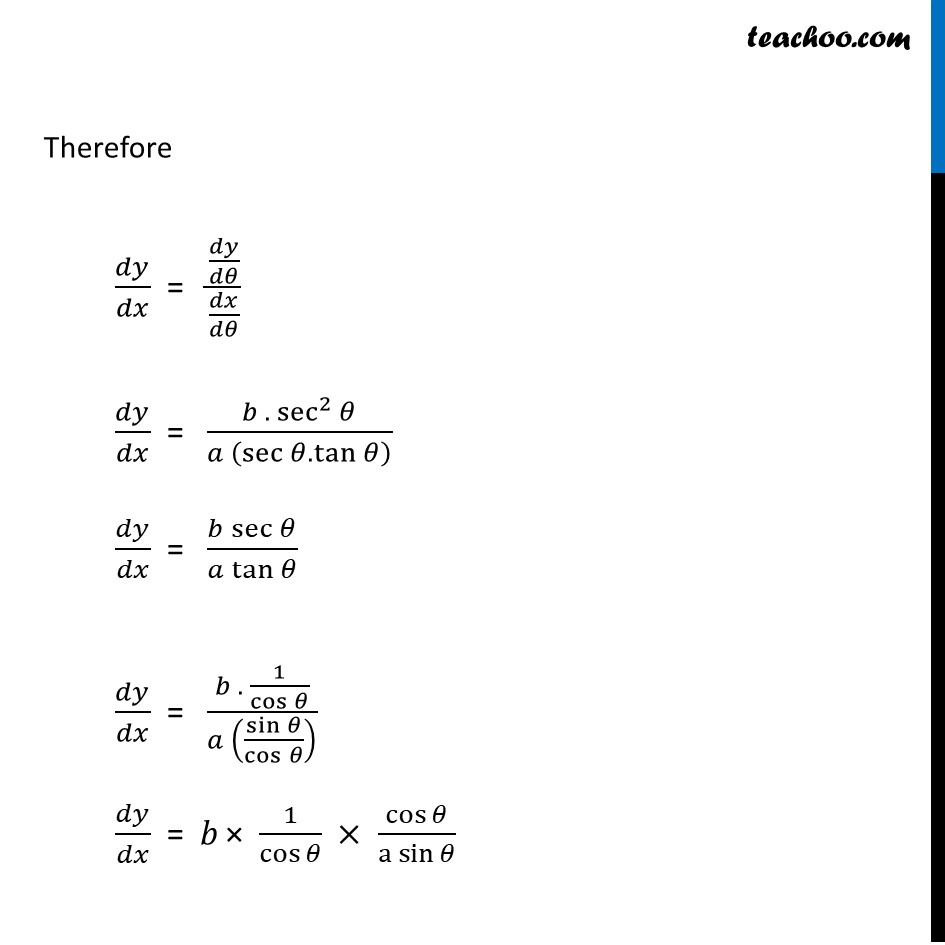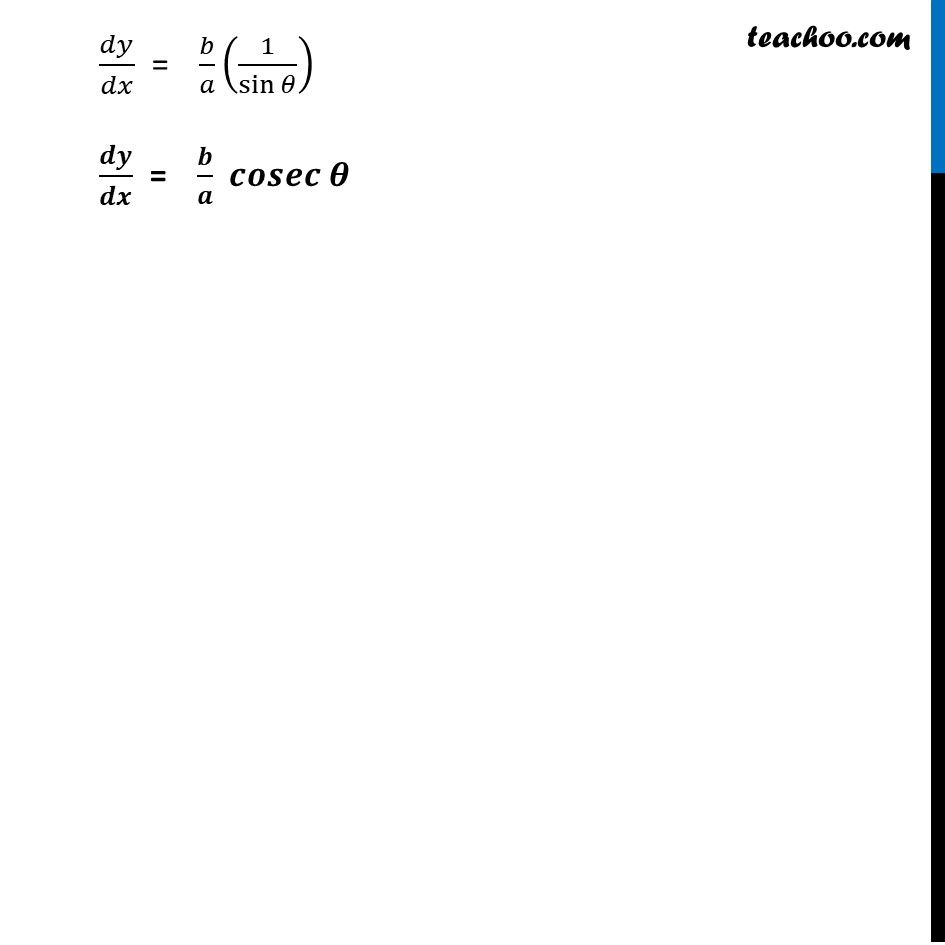Ex 5.6

Chapter 5 Class 12 Continuity and Differentiability
Serial order wiseLearn in your speed, with individual attention - Teachoo Maths 1-on-1 Class

### Transcript

Ex 5.6, 9 If x and y are connected parametrically by the equations without eliminating the parameter, Find 𝑑𝑦/𝑑𝑥, 𝑥=𝑎 sec ⁡𝜃, 𝑦=𝑏 tan⁡𝜃Here 𝑑𝑦/𝑑𝑥 = (𝑑𝑦/𝑑𝜃)/(𝑑𝑥/𝑑𝜃) Calculating 𝒅𝒚/𝒅𝜽 𝑑𝑦/𝑑𝜃 = 𝑑(𝑏 tan⁡𝜃 )/𝑑𝜃 𝑑𝑦/𝑑𝜃 = 𝑏 𝑑(tan⁡𝜃 )/𝑑𝜃 𝑑𝑦/𝑑𝜃 = 𝒃 .〖𝐬𝐞𝐜〗^𝟐⁡𝜽 Calculating 𝒅𝒙/𝒅𝜽 𝑑𝑥/𝑑𝜃 = 𝑑(𝑎 sec ⁡𝜃)/𝑑𝜃 𝑑𝑥/𝑑𝜃 = 𝑎 𝑑(sec ⁡𝜃)/𝑑𝜃 𝑑𝑥/𝑑𝜃 = 𝒂 (𝒔𝒆𝒄⁡𝜽.𝒕𝒂𝒏⁡𝜽 ) Therefore 𝑑𝑦/𝑑𝑥 = (𝑑𝑦/𝑑𝜃)/(𝑑𝑥/𝑑𝜃) 𝑑𝑦/𝑑𝑥 = (𝑏 .〖 sec〗^2⁡𝜃)/(𝑎 (sec⁡𝜃.tan⁡𝜃 ) ) 𝑑𝑦/𝑑𝑥 = (𝑏 sec⁡𝜃)/(𝑎 tan⁡𝜃 ) 𝑑𝑦/𝑑𝑥 = (𝑏 . 1/cos⁡𝜃 )/(𝑎 (sin⁡𝜃/cos⁡𝜃 ) ) 𝑑𝑦/𝑑𝑥 = 𝑏 × 1/cos⁡𝜃 × cos⁡𝜃/〖a sin〗⁡𝜃 𝑑𝑦/𝑑𝑥 = 𝑏/𝑎 (1/sin⁡𝜃 ) 𝒅𝒚/𝒅𝒙 = 𝒃/𝒂 𝒄𝒐𝒔𝒆𝒄 𝜽